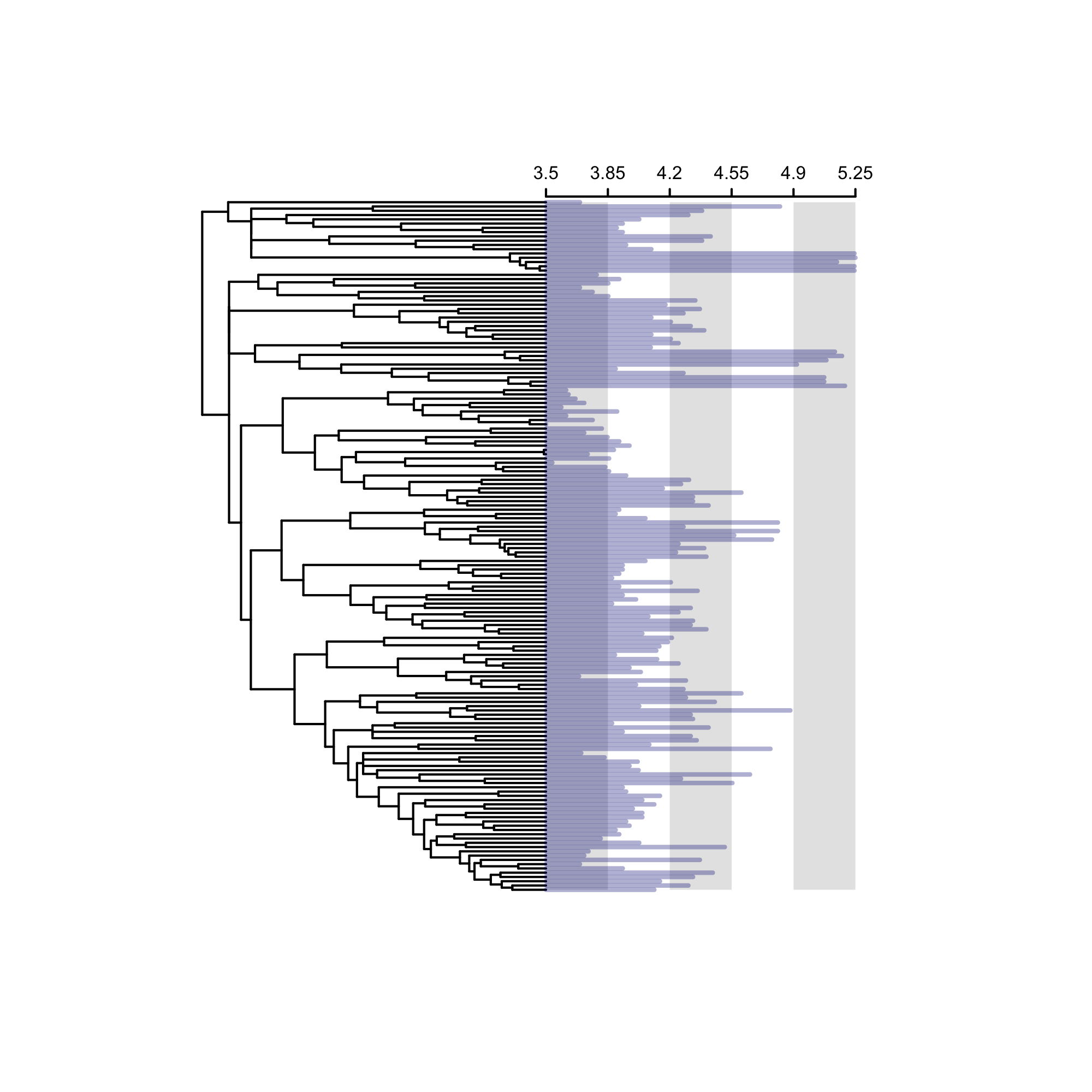Models Of Trait Macroevolution On Trees (MOTMOT) is an R package that allows for testing of models of trait evolution (Thomas et al. 2012).

# Introduction

First we install

``install.packages("motmot")``

``library(motmot)``

For these examples we will use anoles lizard data available from MOTMOT. A time-calibrated phylogeny of Anolis species `anolis.tree`, and various trait and biogeographical trait data `anolis.data`.

``````data(anolis.tree)
data(anolis.data)
attach(anolis.data)
anolis.tree``````
``````##
## Phylogenetic tree with 165 tips and 164 internal nodes.
##
## Tip labels:
##  A_occultus, A_darlingt, A_monticol, A_bahoruco, A_dolichoc, A_henderso, ...
## Node labels:
##  2, 2, 2, 2, 2, 2, ...
##
## Rooted; includes branch lengths.``````

We will use the continuous trait data: male snout-ventral length `Male_SVL`. Here, we construct a matrix of just `Male_SVL` data, remove missing data, and log-transform the values. All this can be done using the function `sortTraitData`

``````sortedData <- sortTraitData(phy = anolis.tree, y = anolis.data,
data.name = "Male_SVL", pass.ultrametric = TRUE)
phy <- sortedData\$phy
male.length <- sortedData\$trait``````

Finally, we will ‘prune’ the species from the tree using `drop.tip` from APE. We plot our tree and data using the MOTMOT `traitData.plot` function.

``````traitData.plot(y = male.length, phy = phy, lwd.traits = 2,
col.label = "#00008050", tck = -0.01, mgp = c(0, 0.2, 0),
cex.axis = 0.5, show.tips = FALSE)``````Figure 1. TraitData showing the realtive male snout-vent length at the tips

For the sake of brevity, in the following examples we fit the models to a subset of these data: including the clade from node 182 only using the APE function `extract.clade`.

``````## uncomment to view the tree
# plot(phy, show.tip.label=FALSE, no.margin=TRUE, edge.col="grey20")
# nodelabels(182, 182, bg="black", col="white")

# Models of trait evolution

We can now test various models of evolution using our trait data.

## Brownian motion

To start we will fit a simple Brownian motion model to the data, as the null hypothesis of phylogenetic trait evolution (Cavalli-Sforza and Edwards 1967; Felsenstein 1973; 1985). Brownian motion describes a process in which tip states are modelled under the assumption of a multi-variate normal distribution. On a phylogeny, the multi-variate mean of tip states is equal to the root state estimate, and variance accummulates linearly through time. Trait evolution is shared but following a split individual branches evolve and accummulate trait variance independently from their shared ancestral value.

The function `transformPhylo.ML` is used to fit Brownian motion models and its derivatives. Here we fit a simple Brownian motion model to the subset of anolis male SVL data to obtain the Brownian variance, ancestral estimate, log-likelihood, Akaike Information Criterion (AIC), and small-sample AIC (AICc).

``````bm.ml <- transformPhylo.ML(phy = phy.clade, y = male.length.clade, model = "bm")
bm.ml``````
``````## \$brownianVariance
##             [,1]
## [1,] 0.001858067
##
## \$logLikelihood
##  -0.2838382
##
## \$root.state
##  3.849481
##
## \$AIC
##  4.567676
##
## \$AICc
##  5.047676
##
## attr(,"class")
##  "bm.ML"``````

## Pagel’s lambda

Here we fit models to test Pagel’s lambda (Pagel 1997; 1999). Pagel’s lambda is a measure of phylogenetic ‘signal’ in which the degree to which shared history of taxa has driven trait distributions at tips. In this model, internal branch lengths are transformed by the lambda parameter value. When the parameter lambda equals 1, branches are transformed by multiplying by 1 and so the model is equal to Brownian motion (high phylogenetic signal). Values of lambda under 1 suggest there has been less influence of shared history on trait values at the tips. Finally, a lambda value of 0 indicates no phylogenetic influence on trait distributions, and is equivalent to a ‘star phylogeny’ with no shared branch lengths.

The maximum likelihood of lambda can be estimated in MOTMOT.

``````lambda.ml <- transformPhylo.ML(phy = phy.clade, y = male.length.clade,
model = "lambda")
lambda.ml``````
``````## \$MaximumLikelihood
##  6.522191
##
## \$Lambda
##      MLLambda   LowerCI   UpperCI
## [1,] 0.836999 0.5259423 0.9742338
##
## \$brownianVariance
##              [,1]
## [1,] 0.0008245375
##
## \$root.state
##  3.853432
##
## \$AIC
##  -7.044383
##
## \$AICc
##  -6.044383
##
## attr(,"class")
##  "lambda.ML"``````

The maximum likelhood estimate of Pagel’s lambda is equal to 0.84.

A new feature in MOTMOT allows for plotting of the likelihood profile for the branch-transformation parameter, in this case Pagel’s lambda using the argument `profilePlot` in `transformPhylo.ML`.

``````lambda.ml <- transformPhylo.ML(phy = phy.clade, y = male.length.clade,
model = "lambda", profilePlot = TRUE)``````